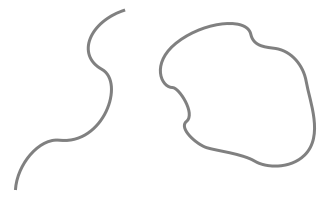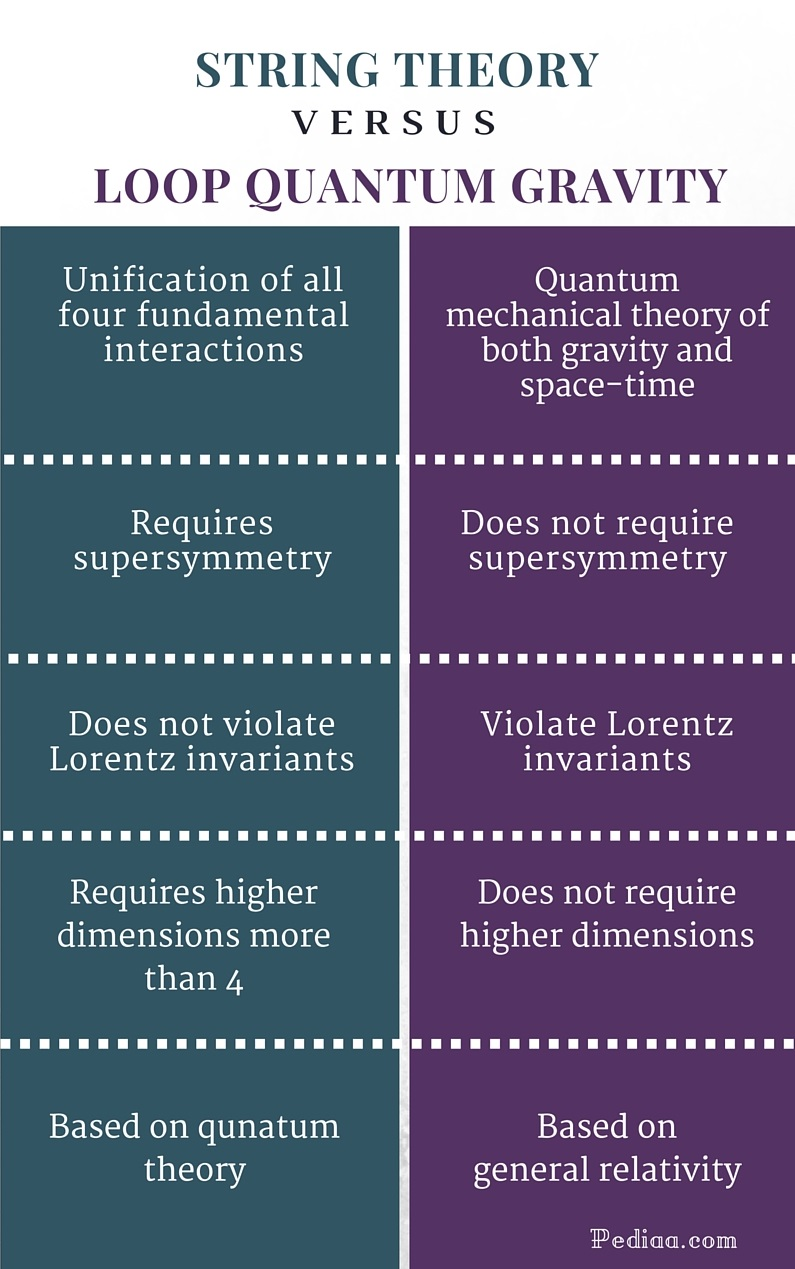# Difference Between String Theory and Loop Quantum Gravity

## Main Difference – String Theory vs Loop Quantum Gravity

String theory and quantum loop gravity are two theories of quantum gravity. But they are two different approaches. String theory is a theoretical attempt to unify all four fundamental interactions. Loop quantum gravity doesn’t attempt to unify fundamental interactions. It is just a theory of quantum gravity. String theory starts from fundamental aspects of quantum theory. Loop quantum gravity, on the other hand, relies on general relativity and quantize gravitational field. String theory works in higher dimensional space- times. But, loop quantum gravity doesn’t require higher dimensions. This is the main difference between string theory and loop quantum gravity. Even though both theories attempt to model a theory of quantum gravity they are theoretically very different. This article attempts to explain fundamental aspects of both theories and the difference between them.

## What is String Theory

String theory is a theoretical attempt to unify all four fundamental interactions into a single unified theory. Several string theories such as superstring theory and M-theory are currently being developed. String theories are developed on the same basic assumptions of quantum theory. String theories start from quantum theory. Quantum theory is a combination of all fundamental interactions except gravity. So, they are based on three fundamental interactions. Eventually, the string theory becomes a unification of all four fundamental interactions.  Thus, string theory is considered to be a theory of quantum gravity.

However, in string theory, the point-like zero-dimensional particles assumed in fundamental particle physics are replaced by one-dimensional string-like objects. These strings are capable of vibrating and stretching. They are the quantum building blocks of matter.

In string theory, the concept of a supersymmetry is essential in order to include fermions. According to the concept of supersymmetry, all fermions must have a superpartner boson. So supersymmetry is a conceptual intermediary that relates bosons (force carriers) and fermions (matter particles). String theories that use the concept of supersymmetry are referred to as theories of superstrings. Normally, string theories require more than four dimensions. In superstring theory, the space-time is considered to be ten- dimensional. In M- theory, space- time is believed to be 11- dimensional.

Basically, string theories are classified by the type of strings assumed in the theory. There are two types of string loops: closed string loops that can part into open string loops and closed string loops that cannot part into open strings. The size of strings is believed to be around Planck length or 10-35m. So, if strings really exist, it would be very difficult to detect with current technologies.

String theory is believed to be a promising candidate for a quantum theory of gravity and is a unification of all four fundamental interaction in nature.Open string and Closed string

## What is Loop Quantum Gravity

Loop quantum gravity is also a theory of quantum gravity. Unlike string theory, loop quantum gravity doesn’t attempt to unify fundamental interactions. Loop quantum gravity simply develops a theory of gravity out of general relativity. It mainly relies on the general relativity and quantizes the gravitational field. Unlike string theory, which focuses mainly on quantum properties of matter, loop quantum gravity mainly focuses on quantum properties of space-time and gravity.

Space- time in loop quantum gravity is viewed as a fabric of loops. So, space isn’t smooth at its original scale rather it is granular. That means, space- time is discrete, and quantized. Mathematically, space- time is a spin network of which the quantum states represent different quantum states of space- time. The fundamental size of space- time fabric lies around the Planck length scale (10-35m) which is the shortest possible distance in physics.

In quantum loop gravity, the infinite singularity appeared at the Big bang is replaced by a big bounce. So, the theory facilitates to study the universe beyond the Big bang. In addition, the theory predicts the entropy of black holes.## Difference Between String Theory and Loop Quantum Gravity

### Unification of Fundamental Interactions:

String theory: It is a unification of all four fundamental interactions.

Loop quantum gravity: It doesn’t attempt to unify fundamental interactions. It is a quantum mechanical theory of both gravity and space-time.

### Supersymmetry:

String theory: It is a very important aspect in order to relate fermions and bosons.

Loop quantum gravity: It doesn’t require a supersymmetry.

### Violation of Lorentz Invariants:

String theory: It doesn’t violate Lorentz invariants.

Loop quantum gravity: It violates Lorentz invariants.

### Dimensions:

String theory: String theory requires higher dimensions more than 4.

Loop quantum gravity: Loop quantum gravity doesn’t require higher dimensions.

### Approach:

String theory: It approaches quantum gravity, assuming main aspects of quantum theory.

Loop quantum gravity: It approaches quantum gravity, assuming main aspects of general relativity.Image Courtesy:

“Open and Closed strings” by Xoneca – Own work (Public Domain) via Commons Wikimedia

“Loop Quantum Theory” by Linfoxman – Foxman (Public Domain) via Commons Wikimedia